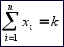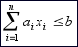# Black Box Scatter Search for General Classes of Binary Optimization Problems

Gortázar, Duarte, Laguna, Martí (2009)
Optsicom project, University of Valencia (Spain)

# Solver Description

BinarySS applies the scatter search (SS) methodology to general classes of binary problems. We focus on optimization problems for which the solutions are represented as binary vectors and that may or may not include constraints. A distinction is made between two sets of general constraint types that are handled directly by the solver and all others constraints that are addressed via penalty functions. In both cases, however, the heuristic treats the objective function evaluation as a black box.

We assume that a problem instance consists of finding a set of values for x = (x1, x2, ..., xn) - where xi = 0 or 1 - in order to maximize an unknown objective function. The user has the choice of specifying whether the problem is unconstrained or constrained. For unconstrained problems, any binary vector of n elements is a feasible solution. If the problem contains constraints the user may choose to transform it into an unconstrained problem by constructing a penalty function whose values are returned by the black box linked to the optimizer. The solver, however, is capable of directly dealing with two general classes of constraints: multiple choice and budget.

Multiple-choice problems are such that k items must be chosen from a total of n. This translates into formulating a multiple-choice constraint that forces that exactly k variables take on the value 1. In mathematical terms:Budget problems are those containing constraints that limit the amount of resources used by a given solution. In this case, resource utilization increases with the number of variables that are set to the value of one. Infeasible solutions occur when the available resources are exceeded. The mathematical form of this constraint may vary because resource utilization may be linear or nonlinear with respect to the variables that take on the value of 1. In the case of the Knapsack problem, for instance, the constraint has the following mathematical form:The budget is given by the value of b and the ai coefficients indicate the amount of resources needed if the ith option is selected. In our implementation, the solution evaluator returns a Boolean value indicating whether the solution is feasible or not. In other words, the solution method does not know the level of infeasibility or what specific variables need to be given a value of zero to make the solution feasible.

# Optimization Problems used for Testing

We have used four combinatorial optimization problems to test our procedure. Solutions to these problems are naturally represented as binary vectors:

1. the max-cut problem
2. the maximum diversity problem
3. the knapsack problem
4. the multi-demand multi-dimensional knapsack problem

The max-cut problem consists of finding a partition of the nodes of a weighted graph into two subsets such that the sum of the weights on the edges connecting the two subsets is maximized. A solution to this problem can be represented as a binary vector with cardinality equal to the number of nodes in the graph (where the value 0 or 1 indicates that the associated node belongs to one or other subset). The max-cut problem falls within the class of binary unconstrained problems.

The maximum diversity problem (MDP) consists of selecting a subset of k elements from a set of n elements in such a way that the sum of the distances between the chosen elements is maximized. Clearly, a solution to this problem can be represented as a binary string x, where variable xi takes on the value of 1 if element i is selected and 0 otherwise, i = 1, ..., n. There is only one constraint in this problem that forces that exactly k variables in the string are assigned the value of 1. This problem belongs to the class of multiple choice problems.

Knapsack problems are well known in the operations research literature. The problem consists of choosing, from a set of items, the subset that maximizes the value of the objective function subject to a capacity constraint. Mathematically, the problem can be expressed as follows:This is a budget-constrained problem that attempts to maximize the benefit associated with selecting a subset of n available objects.

The 0/1 multi-demand multi-dimensional knapsack problem (MDMKP) represents a class of binary problems with general constraints. Mathematically, the problem can be formulated as follows, where the m knapsack constraints are followed by the q demand constraints:# Instances

In this section several instance sets are described. These intance set have been used in the related literature to compare the performance of methods for the problems considered:

• max-cut set: This data set consists of 94 instances from two sources. The Hartmann problems are 10 instances with 125 nodes and 375 edges all with weight values equal to -1 or 1. The second set consists of 84 instances (n=50 to 300) generated with rudy, a machine independent graph generator by Giovanni Rinaldi. The toroidal, planar and random graphs have weights taking the values of -1, 0, or 1. Download.
• mdp set: This data set consists of 92 instances from two sources. The first one with 40 instances, referred to as Glover, for which the values are calculated as the Euclidean distances from randomly generated points with coordinates in the 0 to 100 range. The second one with 52 instances, referred to as Silva, for which the values are integer numbers randomly generated between 50 and 100. The value of n ranges from 50 to 300 and the value of k ranges from 0.1n to 0.3n. Download.
• knapsack set: This data set consists of 96 instances from four types: strongly correlated, subset sum, uncorrelated and weakly correlated instances. We consider 24 instances in each group with n= 100, 300, 1000 and 3000 (6 instance for each n value).Download.
• muti-demand multidimensional knapsack set: This data set consists of 94 instances from Cappanera and Trubian (2005) in which basic multi-knapsack instances were modified by adding demand constraints and also allowing for negative cost coefficients. We consider 47 instances with positive coefficients and 47 with both positive and negative coefficient values. The value of n ranges from 50 to 250, the value of m ranges from 5 to 15 and the value of q ranges from m/2 to m. Download.

# State of the Art Methods

The most relevant commercial solvers for binary problems are:

• Evolutionary Premium Solver by Frontline Systems in the Solver Software Development Kit (version 7.2). This solver manages the constraints with the fcnconstraint function, defining an upper bound in the case of budget problems and lower and upper bounds for multiple-choice problems.
• OptQuest (version 6.2) by OptTek Systems. This engine allows us to define upper requirements to handle constraints in budget problems and dual requeriments for multiple-choice problems.
• Evolver by Palisade Corporation in the Evolver Development Kit (version 4.1.2). This method manages constraints with the evconstraintadd function for both budget and multiple-choice problems.

We also consider state-of-the-art specialized methods for each problem class, all of which are expected to outperform general context-independent solvers. We are only using them as a baseline for comparison. Specifically, we use:

• for the Max-cut instances, we consider the SS method by Martí et al. (2009)
• for the MDP instances we use the SS method by Gallego et al. (2008)
• for the Knapsack instances we use Expknap by Pisinger (1995)
• for the MDMKP instances we use Almha procedure by Arntzen et al. (2006)

# Computational Experience

All the experiments were conducted on a Pentium 4 computer at 3 GHz with 2 GB of RAM. The termination limit was set to 30 seconds, however, some methods finished earlier, as triggered by their internal logic.

We also report the number of instances for which each method is able to find a feasible solution, given that it is not unusual that black-box solvers fail to find feasible solutions for highly constrained instances. Note that in these cases the RPD calculation considers only the feasible solutions that each method is able to find.

To handle multiple constraints in the MDMKP problem we consider the static penalty approach described in Yeniay (2005) in which the value of an infeasible solution x is computed as K(1-s)/m where K is a large positive constant set to 1x109, m is the number of constraints and s is the number of non-violated constraints. We used this penalty function when executing all the methods in the comparison set.